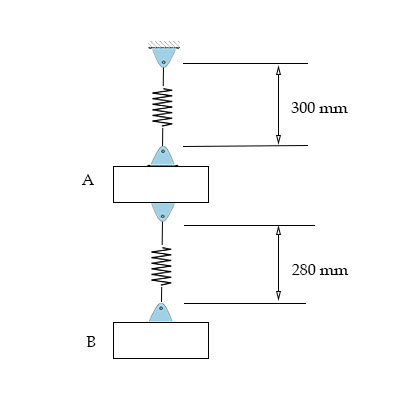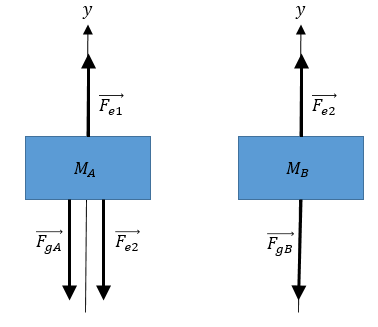# The two springs are identical, with unstretched lengths 250 \ mm and spring constants k=1200 \...

## Question:

The two springs are identical, with unstretched lengths {eq}250 \ mm {/eq} and spring constants {eq}k=1200 \ N/m. {/eq}

a) Draw the free-body diagram of block {eq}A {/eq}.

b) Draw the free-body diagram of block {eq}B {/eq}.

c) What are the masses of the two blocks?## Elastic Force

The elastic force in a spring is proportional to the spring's deformation and its direction opposes that of the deformation. In mathematical form,

{eq}\vec{F}_e=-k\Delta \vec{l} {/eq},

where {eq}k {/eq} is the elastic constant of the spring and {eq}\Delta \vec{x} {/eq} is the deformation vector. This expression is known as Hooke's law.

Become a Study.com member to unlock this answer! Create your account

a-b) The free-body diagram for the two masses are depicted below.The forces acting on mass A are:

{eq}\bullet\; \vec{F}_{gA} {/eq} the...Hooke's Law & the Spring Constant: Definition & Equation

from

Chapter 4 / Lesson 19
201K

After watching this video, you will be able to explain what Hooke's Law is and use the equation for Hooke's Law to solve problems. A short quiz will follow.